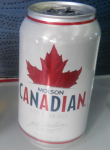# Geography Quiz / Billionaires by Country (2011)

Random Geography or Numbers Quiz

## Can you name the countries with US\$ billionaires in 2011?

#### bythebronze  Plays Quiz not verified by Sporcle

Score
0/55
Timer
05:00
RankCountryBillionaires
1.413
2.115
3.101
4.55
5.52
6.38
7.36
8.32
9.30
10.26
11.25
12.24
13.17
=14.16
=14.16
16.15
=17.14
=17.14
=17.14
20.11
21.10
=22.9
=22.9
=24.8
=24.8
=24.8
=27.6
=27.6
RankCountryBillionaires
=29.5
=29.5
=29.5
=32.4
=32.4
=32.4
=32.4
=32.4
=32.4
=32.4
=39.3
=39.3
=39.3
=39.3
=39.3
=39.3
=39.3
=46.2
=46.2
=46.2
=46.2
=46.2
=46.2
=46.2
=46.2
=54.1
=54.1

### You're not logged in!

Compare scores with friends on all Sporcle quizzes.
OR

## From the Vault See Another

4 to 13 Letter 'E' Places
Luckily, Eyjafjallajökull has 16 letters, so you won't have to spell it today.

### Extras

Created Mar 10, 2011Source
Tags: